# Transistor linearly digitizes airflow

## STMicroelectronics LM393

Steve Woodward

EDN

A sensitive and reliable way to measure airflow is to take advantage of the predictable relationship between heat dissipation and air speed. The principle of thermal anemometry relies on King's Law, which dictates that the power required to maintain a fixed differential between the surface of a heated sensor and the ambient air temperature increases as the square root of air speed. The popular hot-wire anemometer exploits this principle, but it suffers from the disadvantage of using a specialized and fragile metallic filament, the hot wire, as the airflow sensor. The circuit in Figure 1 avoids this disadvantage by using a pair of robust and inexpensive transistors instead of a flimsy wire for air-speed sensing. The Q1/Q2 front end of the circuit borrows from an earlier Design Idea (Reference 1). Just as in the 1996 circuit, the circuit in Figure 1 works by continuously maintaining the condition VQ1 = VQ2. To perform this task, the circuit must keep Q1 approximately 50 °C hotter than Q2.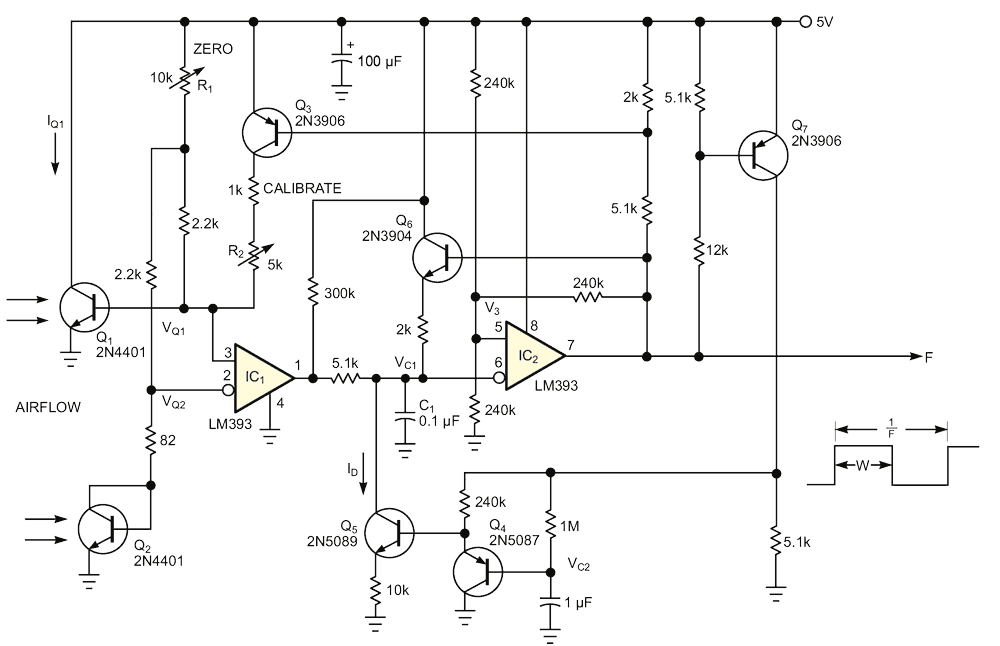Figure 1. Using a simple transistor as sensor, this circuit yields a digitized, linear measurement of air speed.

VBE balance requires this temperature difference, because Q1's collector current, IQ1, is 100 times greater than that of Q2, IQ2. If Q1 and Q2 were at the same temperature, this ratio would result in VQ1's being greater than VQ2 by approximately 100 mV. Proper control of IQ1 establishes differential heating that makes Q1 hotter than Q2. The method thus exploits the approximate –2-mV/°C temperature coefficient of VBE to force VQ balance. The resulting average, IQ1, proportional to the average power dissipated in Q1, is the heat-input measurement that forms the basis for the thermal air-speed measurement. Calibration of the sensor begins with adjustment of the R1 zero-adjust trim. You adjust R1 such that, at zero airflow, VQ1 = VQ2 with no help from Q3. Then, when moving air hits the transistors and increases the heat-loss rate, VQ1 increases and causes comparator IC1 to release the reset on C1. C1 then charges until IC2 turns on, generating a drive pulse to Q1 through Q3.

The resulting squirt of collector current generates a pulse of heating in Q1, driving the transistor's temperature and VBE back toward balance. Proper adjustment of R2 calibrates the magnitude of the IQ1-induced heating pulses to establish an accurate correspondence between pulse rate and air speed.

Now, consider measurement linearization. The square-root relationship of King's Law makes the relationship between heat loss and air speed nonlinear. You must iron the kinks out of the air-speed-calibration curve. You might achieve linearization in software, of course. However, depending on the flexibility of the data system the anemometer works with, a software correction is sometimes inconvenient. Another earlier Design Idea (Reference 2) presented an analog solution to linearization. But if you want the advantages of a digital, pulse-mode output – that is, noise-free transmission over long cable runs – you need a different fix.

The circuit in Figure 1 provides both linearity and a digital output. The average heat the pulses deposit in Q1 is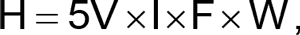where I is the amplitude of the Q1 current pulses (adjusted with R2), F is the output frequency, and W is the pulse width. W is inversely proportional to ID, the discharge current that ramps down VC1 and controls the on-time of IC2. Q4 and Q7 average the output duty cycle to generate a control voltage for Q5 and thus make W a function of F. In fact, the feedback loop this arrangement establishes implicitly makes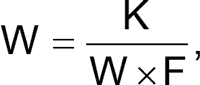where K is a calibration constant determined by the component values. Therefore,and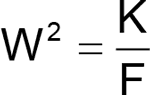This expression yields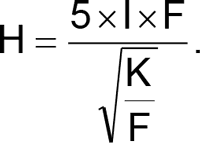making F the desired function of H2 and thus linearizing the relationship between frequency and air speed.

## References

1. Woodward, Steve, "Self-heated transistor digitizes airflow," EDN, March 14, 1996, pg 86.
2. Woodward, Steve, "Transistor and FVCs make linear anemometer," EDN, Sept 26, 1996, pg 72.

## Materials on the topic